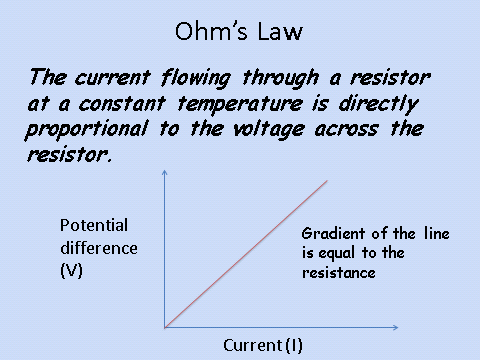# Current Resistance and Potential Difference

## Ohm’s Law

A term must now be introduced which is: Electrical resistance or just resistance.

Resistance is a force that opposes the flow of electric charge, measured in ohms (_Ω)_.

Resistance in a wire is caused by the collision between the electrons (charge carriers) and the ions in the wire.

Ohmic resistors obey Ohm’s law which states:Note: You may have heard potential difference be described as voltage. The exam will always ask using the term potential difference, but you gain credit for using either potential difference or voltage in your answers.

## Ohm’s Law Equation

The equation which you need to recall and apply is:

Potential difference = current x resistance

This can also be written as:

V = I x R

V = potential difference, volts (V)

I = current, amps (A)

R = resistance, ohms (Ω)

The voltage across a lamp is 12V and the current through the lamp is 2A. What is the resistance of the lamp?